CDS  >  CDS I - Mathematics Previous Year Question Paper 2018

# CDS I - Mathematics Previous Year Question Paper 2018 - CDS

Test Description

## 100 Questions MCQ Test CDS ( Combined Defence Services ) Mock Test Series - CDS I - Mathematics Previous Year Question Paper 2018

CDS I - Mathematics Previous Year Question Paper 2018 for CDS 2023 is part of CDS ( Combined Defence Services ) Mock Test Series preparation. The CDS I - Mathematics Previous Year Question Paper 2018 questions and answers have been prepared according to the CDS exam syllabus.The CDS I - Mathematics Previous Year Question Paper 2018 MCQs are made for CDS 2023 Exam. Find important definitions, questions, notes, meanings, examples, exercises, MCQs and online tests for CDS I - Mathematics Previous Year Question Paper 2018 below.
Solutions of CDS I - Mathematics Previous Year Question Paper 2018 questions in English are available as part of our CDS ( Combined Defence Services ) Mock Test Series for CDS & CDS I - Mathematics Previous Year Question Paper 2018 solutions in Hindi for CDS ( Combined Defence Services ) Mock Test Series course. Download more important topics, notes, lectures and mock test series for CDS Exam by signing up for free. Attempt CDS I - Mathematics Previous Year Question Paper 2018 | 100 questions in 120 minutes | Mock test for CDS preparation | Free important questions MCQ to study CDS ( Combined Defence Services ) Mock Test Series for CDS Exam | Download free PDF with solutions
 1 Crore+ students have signed up on EduRev. Have you?
CDS I - Mathematics Previous Year Question Paper 2018 - Question 1

### A man undertakes to do a work in 150 days. He employs 200 men. He finds that only a quarter of the work is done in 50 days. How many additional men should he employ so that the whole work is finished in time?

Detailed Solution for CDS I - Mathematics Previous Year Question Paper 2018 - Question 1

200 men can complete the quarter of work in 50 days

If they continue the work, time taken to complete the remaining work = 3 × 50 = 150 days
[∵ 3/4 th of the work is remaining]

But they have to complete in 100 days to finish in time
Time ∝ 1/Number of employees

Let the number of extra employees required to complete the work in time be x
∴ 150/100 = (200 + x)/200
300 = 200 + x
∴ x = 100

CDS I - Mathematics Previous Year Question Paper 2018 - Question 2

### A train moving with a speed of 60 km per hour crosses an electric pole in 30 seconds. What is the length of train in meters?

Detailed Solution for CDS I - Mathematics Previous Year Question Paper 2018 - Question 2

Speed of the train = 60 km/hr = 60 × 5/18 = 50/3 m/s

Distance travelled by the head of the train after it crosses the pole till the whole train crosses the pole = Length of the train
Distance = Speed × Time
50/3 × 30 = Length of the train
∴ Length of the train = 500 m

CDS I - Mathematics Previous Year Question Paper 2018 - Question 3

### Rs. 120 is distributed among A, B and C so that A’s share is Rs. 20 more than B’s and Rs. 20 less than C’s. What is the B’s share?

Detailed Solution for CDS I - Mathematics Previous Year Question Paper 2018 - Question 3

Let A’s share be Rs. x
∴ B’s share = x - 20 and C’s share = x + 20
Total amount = x + x - 20 + x + 20
120 = 3x
∴ x = 40
B’s share = x - 20 = Rs. 20

CDS I - Mathematics Previous Year Question Paper 2018 - Question 4

In the following table of inverse variations, what are the values of A, B and C respectively?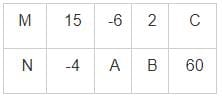Detailed Solution for CDS I - Mathematics Previous Year Question Paper 2018 - Question 4

In inverse variation M × N = constant for all values of M and N

MN = 15 × -4 = -60
-6 × A = -60
∴ A = 10
2 × B = -60
∴ B = -30
C × 60 = -60
∴ C = -1

CDS I - Mathematics Previous Year Question Paper 2018 - Question 5

A person borrows Rs. 5000 at 5% rate of interest per annum and immediately lent it at 5.5%. After two years he collected the amount and settled his loan. What is the amount gained by him this transaction?

Detailed Solution for CDS I - Mathematics Previous Year Question Paper 2018 - Question 5

The extra amount he gains is due the difference in the interest rates.
Difference in the interest rate = 5.5 - 5 = 0.5%
Interest he gained = 5000 × 0.5/100 × 2 = Rs. 50 [∵ Interest = Principal × Rate/100 × Time]

CDS I - Mathematics Previous Year Question Paper 2018 - Question 6

At present the average of the ages of a father and a son is 25 years. After seven years, the son will be 17 years old. What will be the age of father after 10 years?

Detailed Solution for CDS I - Mathematics Previous Year Question Paper 2018 - Question 6

Average age of father and son after 10 years = 25 + 10 = 35
Son’s age after 10 years = Son’s age after 7 years + 3
⇒ 17 + 3 = 20 years
Let the father’s age after 10 years be ‘x’
(x + 20) /2 = 35
x + 20 = 70
∴ x = 50 years

CDS I - Mathematics Previous Year Question Paper 2018 - Question 7

If 5 tractors can plough 5 hectares of land in 5 days, then what is the number of tractor required to plough 100 hectares in 50 days?

Detailed Solution for CDS I - Mathematics Previous Year Question Paper 2018 - Question 7

5 tractors can plough 5 hectares in 5 days
5 tractors can plough 50 hectares in 50 days
1 tractor can plough 10 hectares in 50 days

∴ Number of tractors needed to plough 100 hectares = 100/ (Number of hectares 1 tractor can plough in 50 days)
⇒ 100/10 = 10
∴ 10 tractors can plough 100 hectares in 50 days

CDS I - Mathematics Previous Year Question Paper 2018 - Question 8

A merchant commences with a certain capital and gains annually at the rate of 25%. At the end of 3 years he has Rs. 10,000. What is the original amount that the merchant invested?

Detailed Solution for CDS I - Mathematics Previous Year Question Paper 2018 - Question 8

Let the initial amount invested be Rs x and the amount is compounded at 25% rate

Amount at the end of n years = Principal × (1 + Rate/100)n
10000 = x × (1 + 25/100)3
10000 = x × 1.253
10 × 10 × 10 × 10 = x × 1.25 × 1.25 × 1.25
8 × 8 × 8 × 10 = x [∵ 10/1.25 = 8]
x = Rs. 5120

CDS I - Mathematics Previous Year Question Paper 2018 - Question 9

Which of the following decimal number is a rational number with denominator 37?

Detailed Solution for CDS I - Mathematics Previous Year Question Paper 2018 - Question 9

459 = 17 × 27
Second option is terminating decimal, ∴ denominator has a prime factors of 2 or 5 only

We can evaluate the first decimal and other two decimals are obtained by dividing it by 10 and 100 respectively

Let x = 0.459459459⋯
1000x = 459.459459⋯
1000x = 459 + 0.459459⋯
1000x = 459 + x
∴ 999x = 459
x = 459/999 = (17 × 27) / (37 × 27) = 17/37

∴ 0.459459459⋯ is the decimal with 37 in the denominator when represented as rational number

CDS I - Mathematics Previous Year Question Paper 2018 - Question 10

The annual income of a person decreases by Rs. 64 if the rate of interest decreases from 4% to 3.75%. What is his original annual income?

Detailed Solution for CDS I - Mathematics Previous Year Question Paper 2018 - Question 10

The decrease in the income is due to the reduction in rate of interest.

Decrease in amount = Principal × Reduction in rate of interest/100 × Time
64 = Principal × 0.25/100 × 1
∴ Principal = Rs. 25600

CDS I - Mathematics Previous Year Question Paper 2018 - Question 11

For 0 < m < 1, which one the following is correct?

Detailed Solution for CDS I - Mathematics Previous Year Question Paper 2018 - Question 11

If 0 < m < 1, log10 m is negative so that is the least (All other terms will be positive)

For numbers less than 1, the term with lowest power is the largest
-1 < 1 < 2
∴ m2 < m < m-1
Final order = Log10m < m2 < m < m-1

CDS I - Mathematics Previous Year Question Paper 2018 - Question 12

A gentleman left a sum of Rs. 39000 to be distributed among his widow, 5 sons and 4 daughters. If each son receives three times as much as a daughter receives, and each daughter receives twice as much as their mother receives, then what is the widow’s share?

Detailed Solution for CDS I - Mathematics Previous Year Question Paper 2018 - Question 12

Let the amount received by the widow = Rs x
∴ Amount received by daughter = 2 × x = 2x
Amount received by son = 3 × 2x = 6x

Total amount = Amount received by widow + 5 × Amount received by sons + 4 × Amount received by daughter

39000 = x + 5 × 6x + 4 × 2x
39000 = x + 30x + 8x
39000 = 39x
∴ x = 1000

CDS I - Mathematics Previous Year Question Paper 2018 - Question 13

Three numbers which are co-prime to each other, are such that the product of the first two 286 and that of the last two is 770. What is the sum of three terms?

Detailed Solution for CDS I - Mathematics Previous Year Question Paper 2018 - Question 13

Co-prime numbers have no prime factors in common so when expressed as fraction there will be no simplification

Let the numbers be A, B and C respectively
A × B = 286 and B × C = 770
A × B/ (B × C) = 286/770
A/C = 13/35
∴ A = 13 and C = 35
B = 286/A = 22
Sum of the numbers = 13 + 35 + 22 = 70

CDS I - Mathematics Previous Year Question Paper 2018 - Question 14

The age of a woman is two-digit integer. On reversing this integer, the new integer is the age of her husband who is elder to her. The difference between their ages is one eleventh of their sum. What is the difference between their ages?

Detailed Solution for CDS I - Mathematics Previous Year Question Paper 2018 - Question 14

Let the two digits of the age of the women be y and x (yx)
Age of the women = 10y + x
Age of the men = 10x + y

Difference in their age = 10x + y - 10y - x
⇒ 9x - 9y

Sum of their ages = 10x + y + 10y + x = 11x + 11y
Difference = 1/11 × Sum
9x - 9y = 1/11 × (11x + 11y)
9x - 9y = x + y
8x = 10y

Since x and y can only be natural numbers from 1 to 9, the only possibility is x = 5 and y = 4
Difference in their age = 9x - 9y = 45 - 36 = 9 years

CDS I - Mathematics Previous Year Question Paper 2018 - Question 15

A passenger train and a goods train are running in the same direction on parallel railway tracks. If the passenger train now takes three times as long to pass the goods train, as when they are running in the opposite directions, then what is the ratio of the speed of the passenger train to that of the goods train?

Detailed Solution for CDS I - Mathematics Previous Year Question Paper 2018 - Question 15

For crossing the relative displacement is same and equal to the sum lengths of the trains in both cases

∴ Time ∝ 1/Relative speed

Let the speed of the goods train be x and passenger train be kx, where k is required ratio

Relative speed when moving in opposite direction = kx - (-x) = (k + 1)x [Speeds are added]

Relative speed when moving in same direction = kx - x = (k - 1)x

(Time taken when moving in same direction) / (Time taken when moving in opposite direction) = (Relative Speed in opposite direction) / (Relative Speed in same direction)

3 = (k + 1)x / [(k - 1)x]
3 = (k + 1) / (k - 1)
3k - 3 = k + 1
2k = 4
k = 2
∴ The required ratio = 2 : 1

CDS I - Mathematics Previous Year Question Paper 2018 - Question 16

All odd prime numbers up to 110 are multiplied together. What is the unit digit in this product?

Detailed Solution for CDS I - Mathematics Previous Year Question Paper 2018 - Question 16

Any number multiplied with 5 ends with 5 if it is odd
Product of all odd prime number is an odd number where one of the factor is 5
∴ The unit digit of the product is 5

CDS I - Mathematics Previous Year Question Paper 2018 - Question 17

An alloy A contains two elements, copper and tin in the ratio of 2 : 3, whereas an alloy B contains the same elements in the ratio of 3 : 4. If 20 kg of alloy A, 28 kg of alloy B and some more pure copper are mixed to form a third alloy C which now contains copper and tin in the ratio of 6 : 7, then what is the quantity of the pure copper mixed in the alloy C?

Detailed Solution for CDS I - Mathematics Previous Year Question Paper 2018 - Question 17

Let the amount of pure copper added be ‘x’ kg

Amount of copper in 20 kg of alloy A = 2/ (2 + 3) × 20 = 8 kg

Amount of copper in 28 kg of alloy B = 3/ (3 + 4) × 28 = 12 kg

Total amount of copper in alloy C = 8 + 12 + x = 20 + x

Total amount of alloy C produced = 20 + 28 + x = 48 + x
20 + x = 6/(6 + 7) × (48 + x)
20 + x = 6/13 × (48 + x)
260 + 13x = 288 + 6x
7x = 28
∴ x = 4 kg

CDS I - Mathematics Previous Year Question Paper 2018 - Question 18

A quadratic polynomial ax2 + bx + c = 0 is such that when it is divided by x, (x - 1) and (x + 1), the remainders are 3, 6 and 4 respectively. What is the value of (a + b)?

Detailed Solution for CDS I - Mathematics Previous Year Question Paper 2018 - Question 18

ax2 + bx + c = x (ax + b) + c
Remainder when divided by x = c
∴ c = 3

Let P(x) be a polynomial when divided by (x - a), then the remainder is P(a)

Remainder when divided by (x - 1) = P(1)
6 = a (1) + b(1) + c
6 = a + b + 3
∴ a + b = 3

CDS I - Mathematics Previous Year Question Paper 2018 - Question 19

If the average of 9 consecutive positive integers is 55, then what is the largest integer?

Detailed Solution for CDS I - Mathematics Previous Year Question Paper 2018 - Question 19

The terms are in A.P. where the common difference is 1

For an AP, average is same as the middle most term

Middle most term = 5th term = 55
9th term = 5th term + 4
⇒ 55 + 4 = 59
∴ The largest integer is 59

CDS I - Mathematics Previous Year Question Paper 2018 - Question 20

The average of the ages of 15 students in a class is 19 years. When 5 new students are admitted to the class, the average age of the class becomes 18.5 years. What is the average age of the 5 newly admitted students?

Detailed Solution for CDS I - Mathematics Previous Year Question Paper 2018 - Question 20

Let the average age of 5 students be x
Sum of ages of 15 students + Sum ages of 5 students = Sum of ages of 20 students

Sum = Average × Number of observations
15 × 19 + 5 × x = 20 × 18.5
285 + 5x = 370
5x = 85
∴ x = 17 years

CDS I - Mathematics Previous Year Question Paper 2018 - Question 21

A water tank has been fitted with two taps P and Q and a drain pipe R. Taps P and Q fill at the rate of 12 litres per minute and 10 litres per minute respectively.

Consider the following statements S1, S2 and S3:
S1: Pipe R drains out at the rate of 6 litres per minute
S2: If both the taps and the drain pipe are opened simultaneously, then the tank is filled in 5 hours 45 minutes
S3: Pipe R drains out (fully) the filled tank in 15 hours 20 minutes.
To know what is the capacity of the tank, which one the following is correct?

Detailed Solution for CDS I - Mathematics Previous Year Question Paper 2018 - Question 21

Total volume = Flow rate of R × Time taken to empty

Total volume = (Flow rate of P + Flow rate of Q - Flow rate of R) × Time taken to fill if all the taps and drains are open

To use equation 1, two quantities are required
S1 gives flow rate of R = 6 litres per minute
S3 gives time taken to empty = 15 hours 20 minutes

∴ Volume of tank = 6 × (15 × 60 + 20) = 5520 litres     [∵ 1 hour = 60 minutes]

To use equation 2,
S1: gives the flow rate of R
S2 gives the total time require to fill if all taps and drains are opened.

∴ Volume = (12 + 10 - 6) × (5 × 60 + 45)
⇒ 16 × 345 = 5520 litres
Let the volume of the tank be V litres
By S3: Flow rate of Pipe R = V/ (15 × 60 + 20) = V/920

Substituting in S2
V = (12 + 10 - V/920) × (5 × 60 + 45)
V/345 = 22 - V/920
V/345 + V/920 = 22
168V/57960 + 63V/57960 = 22
231V/57960 = 22
∴ V = 5520 litres
∴ Any two statements are required to find the capacity of the tank

CDS I - Mathematics Previous Year Question Paper 2018 - Question 22

A man can row at a speed of x km/h in still water. If in a stream which is flowing at a speed of y km/h it takes him z hours to row to a place and back, then what is the distance between the two places?

Detailed Solution for CDS I - Mathematics Previous Year Question Paper 2018 - Question 22

Let the distance between the two places be D km
Time taken = Distance/Speed

Speed in upstream = Speed of boat in still water - Speed of the stream = x - y

Speed in downstream = Speed of the boat in still water + Speed of the stream = x + y

Total time taken = Time taken upstream + Time taken downstream

z = D/ (x - y) + D/ (x + y)
z = [D(x + y) + D(x - y)] / [(x - y) (x + y)]
z = 2Dx / (x2 - y2) [∵ (a - b) (a + b) = (a2 - b2)]
∴ D = z(x2 - y2)/2x

CDS I - Mathematics Previous Year Question Paper 2018 - Question 23

A car has an average speed of 60 km per hour while going from Delhi to Agra and has an average speed of y km per hour while returning to Delhi from Agra (by travelling the same distance). If the average speed of the car for the whole journey is 48 km per hour, what is the value of y?

Detailed Solution for CDS I - Mathematics Previous Year Question Paper 2018 - Question 23

Let the distance between Delhi and Agra be D km

Time taken for round journey = D/60 + D/y       [∵ Time = Distance/Speed]
⇒ D(60 + y) / (60y) = 2D/Average speed
⇒ 2 × 60y = Average speed × (60 + y)
120y = 48 × (60 + y)
120y = 2880 + 48y
72y = 2880
∴ y = 40 km/h

CDS I - Mathematics Previous Year Question Paper 2018 - Question 24

An article is sold at a profit of 32%. If the cost price is increased by 20% and the sale price remains the same, then the profit percentage becomes

Detailed Solution for CDS I - Mathematics Previous Year Question Paper 2018 - Question 24

Let the original cost price be Rs ‘x’
Profit = 32% of cost price
∴ Selling price = Cost price + Profit = 1.32x
New cost price = (100 + 20)/100 × x = 1.2x
New profit = Selling price - cost price
⇒ 1.32x - 1.2x = 0.12x
∴ Profit% = 0.12x/1.2x × 100 = 10%

CDS I - Mathematics Previous Year Question Paper 2018 - Question 25

A, B, C, D and E start a partnership firm. Capital contributed by A is three times that contributed by D. E contributes half of A’s contribution, B contributes one third of E’s contribution and C contributes two third of A’s contribution. If the difference between the combined shares of A, D and E and the combined shares of B and C in the total profit of the firm is Rs. 13500, what is the combined share of B, C and E? (The shares are supposed to be proportional to the contributions)

Detailed Solution for CDS I - Mathematics Previous Year Question Paper 2018 - Question 25

Let D’s contribution be x
∴ A’s contribution = 3 × x = 3x
E’s contribution = ½ × 3x = 1.5x
B’s contribution = 1/3 × 1.5x = 0.5x
C’s contribution = 2/3 × 3x = 2x
Total contribution = 3x + 0.5x + 2x + x + 1.5x = 8x
B, C and E contribution = 0.5x + 2x + 1.5x = 4x
Difference between (A + D + E) - (B + C) = 3x + x + 1.5x - 0.5x - 2x = 3x
Share ∝ Contribution
B + C + E contribution/13500 = 4/3
∴ B + C + E contribution = 4/3 × 13500 = Rs. 18000

CDS I - Mathematics Previous Year Question Paper 2018 - Question 26

517 + 518 + 519 + 520 is divisible by

Detailed Solution for CDS I - Mathematics Previous Year Question Paper 2018 - Question 26

517 + 518 + 519 + 520 = 517 × (1 + 5 + 25 + 125)
⇒ 517 × 156 = 517 × 12 × 13
∴ The given expression is divisible by 13

CDS I - Mathematics Previous Year Question Paper 2018 - Question 27

If a + b = 2c, then what is the value of a/(a - c) + c/(b - c)?

Detailed Solution for CDS I - Mathematics Previous Year Question Paper 2018 - Question 27

a + b = 2c
∴ a - c = c - b
a/(a - c) + c/(b - c) = a/(a - c) - c/(a - c) [∵ b - c = -(a - c)]
⇒ (a - c)/(a - c) = 1

CDS I - Mathematics Previous Year Question Paper 2018 - Question 28

If x = y1/a, y = z1/b and z = x1/c where x ≠ 1, y ≠ 1 and z ≠ 1, then what is the value of abc?

Detailed Solution for CDS I - Mathematics Previous Year Question Paper 2018 - Question 28

xa = (y1/a)a = y
(xa)b = yb = (z1/b)b = z
[(xa)b]c = zc = (x1/c)c = x
∴ xabc = x
∴ abc = 1

CDS I - Mathematics Previous Year Question Paper 2018 - Question 29

If 2b = a + c and y2 = xz, then what is xb - c yc - a za - b‑ equal to?

Detailed Solution for CDS I - Mathematics Previous Year Question Paper 2018 - Question 29

2b = a + c
∴ b - c = a - b
xb - c yc - a za - b = xb - c yc - a zb - c
⇒ (xz)b - c yc - a
⇒ (y2)b - c yc - a
⇒ (y)2b - c - a = y0 = 1

CDS I - Mathematics Previous Year Question Paper 2018 - Question 30

Which one of the following is correct?

Detailed Solution for CDS I - Mathematics Previous Year Question Paper 2018 - Question 30

If decimal expansion terminates, the denominator of the rational number is 2 or 5 or combinations for their powers

If the decimal expansion is non terminating but repeating, then it is a rational number with terms in prime factorization of the denominators to be numbers other than 2 or 5

If the decimal expansion is non terminating and non repeating, then it is an irrational number

CDS I - Mathematics Previous Year Question Paper 2018 - Question 31

If the roots of the equation px2 + x + r = 0 are reciprocal to each other, then which one of the following is correct?

Detailed Solution for CDS I - Mathematics Previous Year Question Paper 2018 - Question 31

Products of the roots of quadratic equation = c/a
In this case, product = 1 [∵ Roots are reciprocal of each other]
1 = r/p
∴ p = r

CDS I - Mathematics Previous Year Question Paper 2018 - Question 32

If 65x - 33y = 97 and 33x - 65y = 1, then what is xy equal to?

Detailed Solution for CDS I - Mathematics Previous Year Question Paper 2018 - Question 32

65x - 33y = 97     ----(1)
33x - 65y = 1     ----(2)
Equation 1 + 2
98x - 98y = 98
∴ x - y = 1 or x = y + 1
Substituting in equation 1
65y + 65 - 33y = 97
32y = 32
∴ y = 1 and x = 1 + 1 = 2
∴ xy = 2 × 1 = 2

CDS I - Mathematics Previous Year Question Paper 2018 - Question 33

If b/y + z/c = 1 and c/z + x/a = 1, then what is (ab + xy)/bx equal to?

Detailed Solution for CDS I - Mathematics Previous Year Question Paper 2018 - Question 33

(ab + xy)/bx = a/x + y/b
⇒ (x/a)-1 + (b/y)-1
⇒ (1 - c/z)-1 + (1 - z/c)-1
⇒ z/(z - c) + c/(c - z)
⇒ (z - c) / (z - c) = 1

CDS I - Mathematics Previous Year Question Paper 2018 - Question 34

If (a2 - 1)/a = 5, then what is the value of (a6 - 1)/a3?

Detailed Solution for CDS I - Mathematics Previous Year Question Paper 2018 - Question 34

(a2 - 1)/a = 5
a - 1/a = 5

Cubing on both the sides
a3 - 1/a3 - 3 × a × 1/a × (a - 1/a) = 125 [∵ (a - b)3 = a3 - b3 - 3ab (a - b)]
a3 - 1/a3 - 3 × 5 = 125
∴ a3 - 1/a3 = (a6 - 1)/a3 = 125 + 15 = 140

CDS I - Mathematics Previous Year Question Paper 2018 - Question 35

If x + y + z = 0, then what is (y + z - x)3 + (z + x - y)3 + (x + y -z)3 equal to?

Detailed Solution for CDS I - Mathematics Previous Year Question Paper 2018 - Question 35

(y + z - x)3 + (z + x - y)3 + (x + y -z)3 = (x + y + z - 2x)3 + (y + z + x - 2y)3 + (x + y + z - 2z)3
⇒ (-2x)3 + (-2y)3 + (-2z)3 [∵ x + y + z = 0]
⇒ -8 × (x3 + y3 + z3)
If x + y + z = 0, then x3 + y3 + z3 = 3xyz
∴ The given expression = -8 × 3xyz = -24xyz

CDS I - Mathematics Previous Year Question Paper 2018 - Question 36

If (x + 3) is a factor of x3 + 3x2 + 4x + k, then what is the value of k?

Detailed Solution for CDS I - Mathematics Previous Year Question Paper 2018 - Question 36

If (x - a) is a factor of polynomial P(x), then P(a) = 0
∴ (-3)3 + 3 × (-3)2 + 4 × (-3) + k = 0
-12 + k = 0
k = 12

CDS I - Mathematics Previous Year Question Paper 2018 - Question 37

The smallest integer with 4 digits which is a perfect square is

Detailed Solution for CDS I - Mathematics Previous Year Question Paper 2018 - Question 37

We know that 302 = 900
312 = 961
322 = 1024
∴ 1024 is the smallest 4 digit perfect square

CDS I - Mathematics Previous Year Question Paper 2018 - Question 38

Which one the following is a zero of the polynomial 3x3 + 4x2 - 7 = 0

Detailed Solution for CDS I - Mathematics Previous Year Question Paper 2018 - Question 38

Sum of the coefficients of the polynomial is zero (3 + 4 - 7 = 0)
∴ 1 is the root of the polynomial

CDS I - Mathematics Previous Year Question Paper 2018 - Question 39

There are two numbers which are greater than 21 and their LCM and HCF are 3003 and 21 respectively. What is the sum of these numbers?

Detailed Solution for CDS I - Mathematics Previous Year Question Paper 2018 - Question 39

If their HCF = 21, then the numbers are form 21a and 21b respectively, where a and b are coprimes

LCM × HCF = Product of the numbers
3003 × 21 = 21a × 21b
∴ ab = 143 = 13 × 11 or 143 × 1

Since both the numbers are greater than 21
a = 13 and b = 11

So the numbers are 21 × 13 = 273 and 21 × 11 = 231
Sum of the numbers = 273 + 231 = 504

CDS I - Mathematics Previous Year Question Paper 2018 - Question 40

If α and β are the roots of the equation ax2 + bx + c = 0, then what is the value of the expression (α + 1) (β + 1)?

Detailed Solution for CDS I - Mathematics Previous Year Question Paper 2018 - Question 40

Sum of roots = -b/a
∴ α + β = -b/a
Product of roots = c/a
∴ αβ = c/a
1 + α + β + αβ = 1 - b/a + c/a = (a - b + c)/a
1 + α + β (α + 1) = (a - b + c)/a
(α + 1) (β + 1) = (a - b + c)/a

CDS I - Mathematics Previous Year Question Paper 2018 - Question 41

The remainder when 3x3 + kx2 + 5x - 6 is divided by (x + 1) is -7. What is the value of k?

Detailed Solution for CDS I - Mathematics Previous Year Question Paper 2018 - Question 41

P(a) is the remainder obtained when the polynomial is divided by (x - a)
∴ 3(-1)3 + k(-1)2 + 5(-1) - 6 = -7
k - 14 = -7
∴ k = 7

CDS I - Mathematics Previous Year Question Paper 2018 - Question 42

If f(x) and g(x) are polynomials of degree p and q respectively, then the degree of [f(x) ± g(x)] (if it is non zero) is

Detailed Solution for CDS I - Mathematics Previous Year Question Paper 2018 - Question 42

Degree is the highest power of the given polynomial

If f(x) and g(x) are added the maximum of them will be the degree unless some term cancels.

If p = q, and the coefficients are negative to each other, the first term may get cancelled. Then the degree is less than the maximum.

∴ Degree can either be equal or less than the max(p, q)

CDS I - Mathematics Previous Year Question Paper 2018 - Question 43

What is the value of [(√5 - √3) / (√5 + √3)] - [(√5 + √3) / (√5 - √3)]?

Detailed Solution for CDS I - Mathematics Previous Year Question Paper 2018 - Question 43

(√5 - √3) × (√5 + √3) = 5 - 3 = 2 [∵ (a - b) (a + b) = (a2 - b2)]

(√5 - √3)/2 = 1/(√5 + √3)

∴ [(√5 - √3)/(√5 + √3)] - [(√5 + √3)/(√5 - √3)] = (√5 - √3)2/2 - (√5 + √3)2/2

⇒ [(√5 - √3)2 - (√5 + √3)2]/2

⇒ [2√5 × -2√3]/2 [∵ (a - b) (a + b) = (a2 - b2)]

⇒ -2√15

CDS I - Mathematics Previous Year Question Paper 2018 - Question 44

What is the value of [1/(1 + xb - a + xc - a) + 1/(1 + xa - b + xc - b) + 1/(1 + xa - c + xb - c)] where x ≠ 0?

Detailed Solution for CDS I - Mathematics Previous Year Question Paper 2018 - Question 44

Multiplying numerator and denominator by xa in first term, xb in the second term and xc in third term

The given term = xa/(xa + xb + xc) + xb/(xa + xb + xc) + xc/(xa + xb + xc)
⇒ (xa + xb + xc) / (xa + xb + xc) = 1

CDS I - Mathematics Previous Year Question Paper 2018 - Question 45

The sum of the number and its square is 20. Then the number is

Detailed Solution for CDS I - Mathematics Previous Year Question Paper 2018 - Question 45

Let the number be x
x2 + x = 20
x2 + x - 20 = 0
x2 + 5x - 4x - 20 = 0
x(x + 5) - 4(x + 5) = 0
(x + 5) (x - 4) = 0
∴ x = -5 or 4

CDS I - Mathematics Previous Year Question Paper 2018 - Question 46

If the price of wheat rises by 25%, then by how much percentage must a man reduce his consumption in order to keep his budget the same as before?

Detailed Solution for CDS I - Mathematics Previous Year Question Paper 2018 - Question 46

Let the initial price of wheat and consumptions be x and y respectively

Let the new consumption be y’

Price of the wheat × Consumption = Budget
∴ xy = (100 + 25)/100 × x × y’
xy = 1.25xy’
∴ y’ = y/1.25 = 0.8y
Reduction in consumption = (y - 0.8y)/y × 100 = 20%

CDS I - Mathematics Previous Year Question Paper 2018 - Question 47

1/25 of the students who registered did not appear for the examination, 11/20 of those who appeared passed. If number of registered students is 2000, the number who passed is

Detailed Solution for CDS I - Mathematics Previous Year Question Paper 2018 - Question 47

Number of students who appeared for examination = (1 - 1/25) × 2000 = 1920
Number students who passed = 11/20 × 1920 = 1056

CDS I - Mathematics Previous Year Question Paper 2018 - Question 48

What is the difference between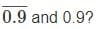Detailed Solution for CDS I - Mathematics Previous Year Question Paper 2018 - Question 48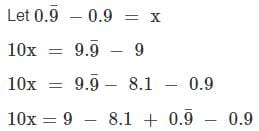10x = 0.9 + x
9x = 0.9
∴ x = 0.1

CDS I - Mathematics Previous Year Question Paper 2018 - Question 49

If A ∶ B = 1 ∶ 2, B ∶ C = 3 ∶ 4, C ∶ D = 2 ∶ 3 and D ∶ E = 3 ∶ 4, then what is B ∶ E equal to?

Detailed Solution for CDS I - Mathematics Previous Year Question Paper 2018 - Question 49

B/E = B/C × C/D × D/E
⇒ ¾ × 2/3 × ¾ = 3/8
∴ B ∶ E = 3 ∶ 8

CDS I - Mathematics Previous Year Question Paper 2018 - Question 50

A work when done by 10 women is completed in 12 days. The same work can be completed in 8 days by 5 men. How many days will it take to complete when 6 women and 3 men are employed to perform the same job?

Detailed Solution for CDS I - Mathematics Previous Year Question Paper 2018 - Question 50

10 women complete work in 12 days
∴ 5 women complete work in 12 × 2 = 24 days
5 men can complete the same work in 8 days
∴ Men are 3 times efficient as women
Three women can be replaced by a man
6 women and 3 men = 6/3 + 3 = 5 men
5 men can complete the work in 8 days
∴ Work is completed in 8 days

CDS I - Mathematics Previous Year Question Paper 2018 - Question 51

The locus of the midpoints of the radii of length 16 cm of a circle is

Detailed Solution for CDS I - Mathematics Previous Year Question Paper 2018 - Question 51

Circle is defined as a locus of all the points at the same distance from the centre.

The distance of all the midpoints of the radii will be at a distance of 8 cm from the centre
∴ It is also a circle but with radius 8 cm

CDS I - Mathematics Previous Year Question Paper 2018 - Question 52

The curved surface area of a right circular cone is 1.76 m2 and its base diameter is 140 cm. What is the height of the cone?

Detailed Solution for CDS I - Mathematics Previous Year Question Paper 2018 - Question 52

Curved surface area of cone = π r l
17600 = 22/7 × 70 × l [∵ 1 m2 = 10000 cm2 and radius = diameter/2]
∴ l = 17600/220 = 80 cm
h = √(l2 - r2)
⇒ √(802 - 702)
⇒ √1500 = 10√15 cm

CDS I - Mathematics Previous Year Question Paper 2018 - Question 53

Consider the following statements:

1. The orthocenter of a triangle always lie inside the triangle
2. The centroid of a triangle always lie inside the triangle
3. The orthocenter of the right angled triangle lies on the triangle
4. The centroid of a right angled triangle lies on the triangle

Q. Which of the above statements are correct?

Detailed Solution for CDS I - Mathematics Previous Year Question Paper 2018 - Question 53

Statement 1:
The orthocenter of an obtuse angled triangle lies outside the triangle

∴ It is false

Statement 2:
Centroid is the intersection point of all medians and all medians are necessarily lying inside the triangle, centroid lies inside the triangle

∴ Statement 2 is true

Statement 3:
Orthocenter is the intersection of altitudes. Since in a right triangle both the sides (except hypotenuse) all altitudes, their point of intersection lies on the triangle.

∴ Statement 3 is true

Statement 4:
If statement 2 is true, statement 4 is false
∴ Statements 2 and 3 are correct

CDS I - Mathematics Previous Year Question Paper 2018 - Question 54

The locus of a point equidistant from two intersecting lines is

Detailed Solution for CDS I - Mathematics Previous Year Question Paper 2018 - Question 54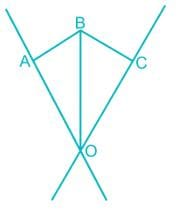Let the intersection point be O and the point which is equidistant from the two lines be B
In triangle AOB and COB
AB = CB [∵ The point is equidistant]
OB is common

∠BAO = ∠BCO = 90° [Distance is measured along perpendicular]
∴ AOB ≅ COB
∠AOB = ∠COB

∴ The locus of all the points at the equidistant from the lines is the angular bisector of the lines

But since there are two pairs of vertically opposite angles made, there will two such angle bisector
∴ Locus is a pair of straight lines

CDS I - Mathematics Previous Year Question Paper 2018 - Question 55

Consider the following statements:

Two triangles are said to be congruent, if
1. Three angles of one triangle are equal to corresponding three angles of the other triangle
2. Three sides of one triangle are equal to the corresponding three sides of the triangle
3. Two sides and the included angle of one triangle are equal to the corresponding two sides and the included of the other triangle
4. Two angles and the included side of one triangle are equal to the corresponding two angles and the included sides of other triangle

Q. Which of the above statements are correct?

Detailed Solution for CDS I - Mathematics Previous Year Question Paper 2018 - Question 55

For congruency, all sides and all angles need to be equal to the corresponding sides and angles

When fixing the angle, the ratio of sides get fixed but while fixing the sides the angle gets fixed.

Statement 1 is false, because only the ratio of sides is fixed and the sides can be expanded and shrunk without changing angle. For example, all equilateral triangles have same angle, but different sides

Statement 2 is true, since fixing sides fix angles. (Cosine formula for triangle)

[SSS congruency]
Statement 3 is true:

With two sides and an included angle, third side is fixed [Cosine formula]

When all sides are fixed, then angles are also fixed.

[SAS congruency]
Statement 4 is true:

When two angles are equal, third angle is also equal [Property sum of angles of triangle]

So the ratios of the sides are equal

Since one of the sides is equal and the ratios are equal, all sides are equal
ASA congruency

CDS I - Mathematics Previous Year Question Paper 2018 - Question 56

Given that the angles of a polygon are all equal and each angle is a right angle.

Statement 1: The polygon has exactly four sides
Statement 2: The sum of the angles of a polygon having n sides is (3n - 8) right angles.

Q. Which one of the following is correct in respect of the above statements?

Detailed Solution for CDS I - Mathematics Previous Year Question Paper 2018 - Question 56

Statement 2:
Sum of interior angles of a polygon = (n - 2) × 180 = (2n - 4) × 90

∴ Statement 2 is false

Statement 1:
Sum of interior angles of the polygon = n × 90° [∵ All angles are right angles]
(2n - 4) × 90 = n × 90
2n - 4 - n
∴ n = 4.
Statement 1 is true

CDS I - Mathematics Previous Year Question Paper 2018 - Question 57

If the length of a side of a square increased by 8 cm, its area increased by 120 square cm. What is the length of a side of the square?

Detailed Solution for CDS I - Mathematics Previous Year Question Paper 2018 - Question 57

Let the original length of the side of the square be x cm

Area = side2
If the length increases by 8cm, area increased by 120 cm2
(x + 8)2 - x2 = 120
x2 + 16x + 64 - x2 = 120 [∵ (a + b)2 = a2 + 2ab + b2]
16x = 56
∴ x = 3.5 cm

CDS I - Mathematics Previous Year Question Paper 2018 - Question 58

What is the largest power of 10 that divides the product 1 × 2 × 3 × 4⋯ × 23 × 24 × 25?

Detailed Solution for CDS I - Mathematics Previous Year Question Paper 2018 - Question 58

10 = 2 × 5, i.e. Both 2 and 5 have to be available to make it a multiple of 10

Since number of multiples of 2 is greater than the number of multiples of 5 in the interval, Number of multiples of 10 = Number of multiples of 5

Number of multiples of 5 = 5, 10, 15, 20, 25

But 25 = 5 × 5
∴ Power of 5 in the prime factorization of the given term = 6
∴ Largest power of 10 that divides the given product = 6

CDS I - Mathematics Previous Year Question Paper 2018 - Question 59

Walls (excluding their roofs and floors) of 5 identical rooms having length, breadth and height 6m, 4 m and 2.5 m respectively are to be painted. Paints are available only in cans of 1 L and one litre of paint can be used for painting 20 square metres. What is the number of cans required for painting?

Detailed Solution for CDS I - Mathematics Previous Year Question Paper 2018 - Question 59

Area to be painted in each room = 2 (lh + bh)
⇒ 2 × (6 × 2.5 + 4 × 2.5) = 50 m2

Total area to be painted = 5 × 50 = 250 m2 [∵ 5 rooms are to be painted]

Amount of paint required in litres = 250/20 = 12.5 litres [∵ 1 L paints 20 m2]

If one can contains 1 L, 13 cans have to be purchased to get 12.5 litres [∵ Fraction is not possible]

CDS I - Mathematics Previous Year Question Paper 2018 - Question 60

A rectangular pathway having width 4.5 m and length 10 m will have to be tiled using square tiles of side 50 cm. Each packet of such tiles contains 20 pieces and costs Rs. 100. What will be the total cost of tiles for the pathway?

Detailed Solution for CDS I - Mathematics Previous Year Question Paper 2018 - Question 60

Number of tiles in a row = Width/Side of square
⇒ 4.5/0.5 = 9 tiles [∵ 50cm = 0.5 m]

Number of tiles in a column = Length/Side of the square
⇒ 10/0.5 = 20 tiles
Total number of tiles required = 9 × 20
Number of packets required = 9 × 20/20 = 9 packets
Cost of nine packets = 9 × 100 = Rs. 900

CDS I - Mathematics Previous Year Question Paper 2018 - Question 61

A cube of maximum value (each corner touching the surface from inside) is cut from a sphere. What is the ratio of volume of the cube to that of the sphere?

Detailed Solution for CDS I - Mathematics Previous Year Question Paper 2018 - Question 61

When cube of maximum volume is cut from sphere, the diagonal of the cube = Diameter of the sphere
∴ √3 a = 2r
a/r = 2/√3
Volume of cube/Volume of sphere = a3/ (4/3 π r3)
⇒ 3/(4π) × (a/r)3
⇒ 3/(4π) × (2/√3)3
⇒ 2/(√3π)
∴ The required ratio = 2 ∶ √3π

CDS I - Mathematics Previous Year Question Paper 2018 - Question 62

If the ratio of the circumference of the base of a right circular cone of radius r to its height is 3 : 1, then what is the area of the curved surface of the cone?

Detailed Solution for CDS I - Mathematics Previous Year Question Paper 2018 - Question 62

Given 2πr/h = 3/1
∴ r : h = 3 : 2π
l = √(r2 + h2)
⇒ r × √[1 + (h/r)2]
⇒ r × √[1 + (2π/3)2] = r/3 × √(4π2 + 9)
Curved surface area of cone = πrl
⇒ πr × r/3 × √(4π2 + 9)
⇒ [πr2 √(4π2 + 9)]/3

CDS I - Mathematics Previous Year Question Paper 2018 - Question 63

A wire is in the form of a circle of radius 98 cm. A square is formed out of the wire. What is the length of a side of the square? (Use π = 22/7)

Detailed Solution for CDS I - Mathematics Previous Year Question Paper 2018 - Question 63

Circumference of the circle = Perimeter of the square
2πr = 4s
∴ s = πr/2
⇒ 22/7 × 98/2 = 154 cm

CDS I - Mathematics Previous Year Question Paper 2018 - Question 64

Directions: Consider the following:

In a triangle ABC, a, b and c are the lengths of the sides and p, q and r are the lengths of its medians.

Q. Which one of the following is correct?

Detailed Solution for CDS I - Mathematics Previous Year Question Paper 2018 - Question 64Sum of two sides of a triangle > third side
In triangle ABD,
AB + BD > AD       ---- 1
In triangle ACD
AC + CD > AD        ---- 2
Adding the inequalities 1 and 2
AB + BD + CD + AC > 2AD
AB + BC + AC > 2 AD
a + b + c > 2p
Similarly for other medians
a + b + c > 2q
a + b + c > 2r
3(a + b + c) > 2(p + q + r)

CDS I - Mathematics Previous Year Question Paper 2018 - Question 65

In a triangle ABC, a, b and c are the lengths of the sides and p, q and r are the lengths of its medians.

Q. Which of the following is correct?

Detailed Solution for CDS I - Mathematics Previous Year Question Paper 2018 - Question 65

Let the centroid be O
In triangle AOB
AO + BO > AB
Centroid divides the median in the ratio 2 ∶ 1
∴ 2p/3 + 2q/3 > c
Similarly for other medians
2q/3 + 2r/3 > a
2p/3 + 2r/3 > b
4/3(p + q + r) > (a + b + c)
∴ 4(p + q + r) > 3(a + b + c)

CDS I - Mathematics Previous Year Question Paper 2018 - Question 66

What is the area of the largest circular disc cut from a square of side 2/√π units?

Detailed Solution for CDS I - Mathematics Previous Year Question Paper 2018 - Question 66

Side of the square = Diameter of the circle
∴ Radius of the circle, r = 2/√π × ½ = 1/√π
Area of the circle = π r2
⇒ π × 1/√π × 1/√π = 1 square units

CDS I - Mathematics Previous Year Question Paper 2018 - Question 67

The product of the lengths of the diagonals of a square is 50 square units. What is the length of a side of the square?

Detailed Solution for CDS I - Mathematics Previous Year Question Paper 2018 - Question 67

Both the diagonals have same length,
∴ Product of length of diagonals = Square of the length of diagonal
Length of diagonal = √2 × length of side
Length of diagonal2 = 2 × Length of side2
50 = 2 × s2
25 = s2
∴ s = 5 units

CDS I - Mathematics Previous Year Question Paper 2018 - Question 68

The surface area of a closed cylindrical box is 352 square cm. If its height is 10cm, then what is its diameter? (Use π = 22/7)

Detailed Solution for CDS I - Mathematics Previous Year Question Paper 2018 - Question 68

TSA of cylinder = 2πr(h + r)
352 = 2 × 22/7 × r (10 + r)
56 = r (10 + r)
4 × 14 = r × (10 + r)
∴ r = 4 cm
Diameter = 2 × radius = 8 cm

CDS I - Mathematics Previous Year Question Paper 2018 - Question 69

A square and an equilateral triangle have the same perimeter, If the diagonal of the square is 6√2 cm, then what is the area of the triangle?

Detailed Solution for CDS I - Mathematics Previous Year Question Paper 2018 - Question 69

Diagonal of the square = √2 × side of the square
∴ Side of the square = 6√2/√2 = 6 cm
4 × Side of the square = 3 × Side of the triangle
4 × 6 = 3 × Side of the triangle
∴ Side of the equilateral triangle = 8 cm
Area of the equilateral triangle = √3/4 × side2
⇒ √3/4 × 82 = 16√3 cm2

CDS I - Mathematics Previous Year Question Paper 2018 - Question 70

What is the area of the region bounded internally by a square of side of length ‘a’ and externally by a circle passing through the four corners of the square?

Detailed Solution for CDS I - Mathematics Previous Year Question Paper 2018 - Question 70

Diagonal of the square = Diameter of the circle
√2 a = 2r
∴ r = a/√2

Area of the required region = Area of the circle - Area of the square
⇒ πr2 - a2
⇒ π (a/√2)2 - a2 = (π - 2)a2/2 square units

CDS I - Mathematics Previous Year Question Paper 2018 - Question 71

In the figure given below, XA and XB are two tangents to a circle. If ∠AXB = 50° and AC is parallel to XB, then what is ∠ ACB equal to?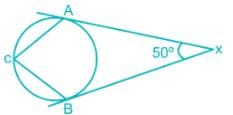Detailed Solution for CDS I - Mathematics Previous Year Question Paper 2018 - Question 71

Let O be the centre of the circle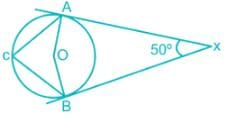∠A + ∠O + ∠B + ∠X = 360° [Angle sum property of quadrilateral]

90° + ∠O + 90° + 50° = 360° [∵ Tangent is perpendicular to the radius]
∴ ∠AOB = 130°
∠ACB = ½ × 130 = 65° [∵ Angle subtended by a chord at any point on the circle is half the angle subtended at centre]

CDS I - Mathematics Previous Year Question Paper 2018 - Question 72

In the figure given below, p, q, r are parallel lines; l and m are two transversals.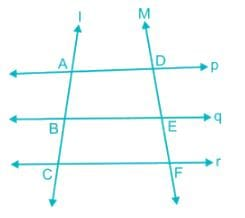Consider the following:
1. AB : AC = DE : DF
2. AB × EF = BC × DE

Q. Which of the above is/are correct?

Detailed Solution for CDS I - Mathematics Previous Year Question Paper 2018 - Question 72

Construct a perpendicular from A to the line CF, Let it meet BE at O and CF at P

Similarly a perpendicular from D is drawn to the line CF, let it meet BE and CF at R and S

In triangle ABO and ACP
∠A is common and ∠O = ∠P = 90°
ABO ∼ ACP
∴ AB/AC = AO/AP
In triangle DRE and DSF
∠D is common and ∠R = ∠S = 90°
DRE ∼ DSF
∴ DE/DF = DR/DS
Parallel lines are equally spaced
∴ AO = DR and AP = DS
∴ AB/AC = DE/DF
So statement 1 is correct
AB/ (AC - AB) = DE/ (DF - DE)
AB/BC = DE/EF
∴ AB × EF = BC × DE
So statement 2 is also correct

CDS I - Mathematics Previous Year Question Paper 2018 - Question 73

In the equilateral triangle ABC given below, AD = DB and AD = EC. If l is the length of a side of the triangle, then what is the area of the shaded region?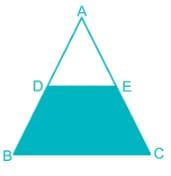Detailed Solution for CDS I - Mathematics Previous Year Question Paper 2018 - Question 73

∠A is common
∴ Area(ADE) = (1/2)2 × Area(ABC)
⇒ ¼ × √3/4 × l2 = √3/16 × l2 [∵ Area of equilateral triangle = √3/4 × l2]
Area of shared region = Area(ABC) - Area(ADC)
⇒ √3/4 × l2 - √3/16 × l2
⇒ 3√3/16 × l2

CDS I - Mathematics Previous Year Question Paper 2018 - Question 74

In the figure given below, SPT is a tangent to the circle at P and O is the centre of the circle. If ∠QPT = α, then what is ∠ POQ equal to?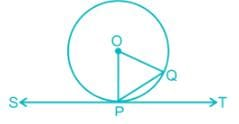Detailed Solution for CDS I - Mathematics Previous Year Question Paper 2018 - Question 74

∠OPT = 90° [∵ Radius is perpendicular to tangent]
∠OPQ = 90° - ∠QPT = 90° - α
∠OQP = 90° - α [∵ OQ = OP]
In triangle OQP
∠O + ∠Q + ∠P = 180°
∠O + 90 - α + 90 - α = 180
∴ ∠O = 2α

CDS I - Mathematics Previous Year Question Paper 2018 - Question 75

In the figure given below, two equal chords cut at point P. If AB = CD = 10 cm, OC = 13 cm (O is the centre of the circle) and PB = 3 cm, then what is the length of OP?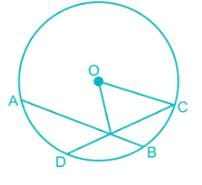Detailed Solution for CDS I - Mathematics Previous Year Question Paper 2018 - Question 75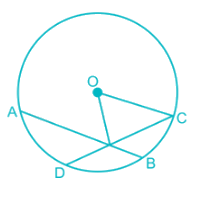Construct OE perpendicular to AB
EB = ½ × AB = 5 cm [∵ Perpendicular from the centre bisects the chord]
In triangle OEB
OB2 = OE2 + EB2
132 = OE2 + 52
∴ OE = 12 cm
In triangle OEP
OP2 = OE2 + EP2
EP = EB - PB = 5 - 3 = 2 cm
OP2 = 122 + 22 = 148
∴ OP = 2√37 cm

CDS I - Mathematics Previous Year Question Paper 2018 - Question 76

A pie chart is drawn for the following data: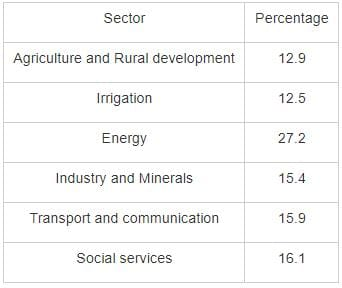Q. What is the angle (approximately) subtended by the social services sector at the centre of the circle?

Detailed Solution for CDS I - Mathematics Previous Year Question Paper 2018 - Question 76

Angle = Percentage of the sector/100 × 360°
⇒ 16.1/100 × 360 = 57.96° ≈ 58°

CDS I - Mathematics Previous Year Question Paper 2018 - Question 77

The arithmetic mean of two numbers is 10 and their geometric mean is 8. What are the two numbers?

Detailed Solution for CDS I - Mathematics Previous Year Question Paper 2018 - Question 77

Let the numbers be a and be respectively
(a + b)/2 = 10
∴ a + b = 20      ----1
√(ab) = 8
∴ ab = 64
(a - b) = √[(a + b)2 - 4ab]
⇒ √(202 - 4 × 64) = √(400 - 256) = 12
∴ a - b = 12      ----2
2a = 32
∴ a = 16 and b = 20 - 16 = 4
∴ The numbers are 16 and 4

CDS I - Mathematics Previous Year Question Paper 2018 - Question 78

The arithmetic mean of 11 observations is 11. The arithmetic mean of the first 6 observations is 10.5 and the arithmetic mean of the last 6 observations is 11.5. What is the sixth observation?

Detailed Solution for CDS I - Mathematics Previous Year Question Paper 2018 - Question 78

Sum of numbers = Average of the numbers × Number of observations

Sum of 11 observations = Sum of first 6 numbers + Sum of last 6 number - 6th number [∵ 6th observation is added twice]
11 × 11 = 10.5 × 6 + 11.5 × 6 - 6th number
121 = 63 + 69 - 6th number
∴ 6th number = 132 - 121 = 11

CDS I - Mathematics Previous Year Question Paper 2018 - Question 79

What is sin4θ - cos4θ equal to for any real number θ?

Detailed Solution for CDS I - Mathematics Previous Year Question Paper 2018 - Question 79

sin2 θ = 1 - cos2 θ
∴ sin4θ - cos4 θ = (1 - cos2θ)2 - cos4 θ
⇒ 1 - 2cos2 θ + cos4 θ - cos4 θ [∵ (a - b)2 = a2 - 2ab + b2]
⇒ 1 - 2cos2 θ

CDS I - Mathematics Previous Year Question Paper 2018 - Question 80

What is cot 1° cot 23° cot 45° cot 67° cot 89° equal to?

Detailed Solution for CDS I - Mathematics Previous Year Question Paper 2018 - Question 80

cot 1° cot 23° cot 45° cot 67° cot 89° = cot 1° cot 23° cot 45° cot (90 - 23°) cot (90 - 1)°
⇒ cot 1° cot 23° cot 45° tan 23° tan 1° [∵ cot (90 - A) = tan A]
⇒ (cot 1° tan 1°) × (cot 23° tan 23°) × cot 45°
⇒ 1 × 1 × 1 [∵ cot A tan A = 1 and cot 45° = 1]
⇒ 1

CDS I - Mathematics Previous Year Question Paper 2018 - Question 81

What angle does the hour hand of a clock describe in 10 minutes of time?

Detailed Solution for CDS I - Mathematics Previous Year Question Paper 2018 - Question 81

Hour hand completes one full revolution in 12 hours
∴ Angle described in 1 hour = 360°/12 = 30°
Angle described in 60 minutes = 30° [∵ 1 hour = 60 minutes]
∴ Angle described in 10 minutes = 30/60 × 10 = 5°

CDS I - Mathematics Previous Year Question Paper 2018 - Question 82

Consider the following statements:
1. (sec2 θ - 1) (1 - cosec2 θ) = 1
2. sin θ (1 + cos θ)-1 + (1 + cos θ) (sin θ)-1 = 2 cosec θ

Q. Which of the above is/are correct?

Detailed Solution for CDS I - Mathematics Previous Year Question Paper 2018 - Question 82

Statement 1:
Sec2 θ - 1 = tan2 θ and cosec2 θ - 1 = cot2 θ

∴ (sec2 θ - 1) (1 - cosec2 θ) = tanθ (-cot2 θ) = -1 [∵ tan θ × cot θ = 1]

∴ Statement 1 is wrong

Statement 2:
sin θ/(1 + cos θ) + (1 + cos θ)/sinθ = [sinθ + (1 + cos θ)2]/[(1 +cos θ) sinθ]

⇒ [sin2 θ + 1 +2cos θ + cosθ]/[(1 +cos θ) sinθ] [∵ (a + b)2 = a2 + 2ab + b2]
⇒ [1 + 1 + 2cos θ]/[(1 + cos θ) sinθ] [∵ sinθ + cosθ = 1]
⇒ [2 + 2cos θ]/[(1 + cos θ) sinθ]
⇒ 2 [1 + cosθ]/[(1 + cos θ) sinθ]
⇒ 2/sinθ = 2 cosecθ [∵ 1/sin θ = cosec θ]
Statement 2 is correct

CDS I - Mathematics Previous Year Question Paper 2018 - Question 83

Each side of a square subtends an angle of 60° at the tip of a tower of height h meters standing at the centre of the square. If l is the length of each side of the square, then what is h2 equal to?

Detailed Solution for CDS I - Mathematics Previous Year Question Paper 2018 - Question 83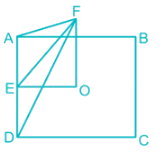EO = l/2 [∵ O is the centre of the square]
∠AFD = 60°

AF = DF due to symmetry and also ∠AFD = 60°
∴ AFD is an equilateral triangle of side l
FE = Altitude of an equilateral triangle = √3l/2
In triangle EFO
FO2 = EF2 - EO2 [∵ Pythagoras theorem]
∴ h2 = (√3l/2)2 - (l/2)2 = l2/2

CDS I - Mathematics Previous Year Question Paper 2018 - Question 84

From a height of h units, a man observes the angle of elevation as α and angle of depression as β of the top and bottom respectively of a tower of height H (> 4h). To what further height should be climb so that the values of angle of elevation and angle of depression get interchanged for the top and bottom of the tower?

Detailed Solution for CDS I - Mathematics Previous Year Question Paper 2018 - Question 84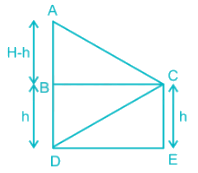∠ACB = α and ∠BCD = β initially
In triangle BCD, cot β = BC/BD
∴ BC = BD cot β = h cot β
In triangle ABC, tan α = AB/BC
∴ AB = BC tan α
H - h = h cotβ × tan α      ----1
∴ H = h (cotβ tan α + 1)      ----2

Now height changes to h’ and the angles get interchanged
∴ H = h’ (cot α tanβ + 1)      ----3

Equating the RHS of equations 2 and 3

h(cotβ tan α + 1) = h’(cot α tan β + 1)

h (1/tanβ × tanα + 1) = h’ (1/tan α × tan β + 1) [∵ tan A = 1/cot A]

h (tan α + tan β)/tan β = h’ (tan α + tan β)/tan α
∴ h’ = h/tan β × tan α

⇒ h cot β × tan α = H - h [From equation 1]
∴ h’ = H - h

Increase in height = h’ - h = H - h - h = H - 2h units

Shortcut:

Since the distance between the building does not change [BC]

If the angle interchanges corresponding height portion of the tallest building should be interchanged

Length of AB in original case = Length of BD in new case

H - h = h’
∴ h’ - h = H - 2h units

CDS I - Mathematics Previous Year Question Paper 2018 - Question 85

If sec x cosec x = 2, then what is the tannx + cotnx equal to?

Detailed Solution for CDS I - Mathematics Previous Year Question Paper 2018 - Question 85

Secx cosecx = 2
1/cosx × 1/sinx = 2
∴ sinx cosx = ½
(sinx - cosx)2 = sin2x + cos2x - 2sinx cosx
⇒ 1 - 2 × ½ = 0 [∵ sin2x + cos2x = 1]
∴ sinx = cos x
∴ sinx/cosx = 1
tanx = 1, cotx = 1/tanx = 1
∴ tannx + cotnx = 1n + 1n = 2

CDS I - Mathematics Previous Year Question Paper 2018 - Question 86

If cos x + cos2 x = 1, then what is sin2 x + sin4 x equal to?

Detailed Solution for CDS I - Mathematics Previous Year Question Paper 2018 - Question 86

cos x + cos2 x = 1
cos x = 1 – cos2 x
cos x = sin2 x [∵ 1 – cos2 A = sin2 A]
Substituting cos x = sin2 x in the main equation
sin2 x + sin4 x = 1

CDS I - Mathematics Previous Year Question Paper 2018 - Question 87

If sin A + cos A = p and sin3A + cos3A = q, then which one of the following is correct?

Detailed Solution for CDS I - Mathematics Previous Year Question Paper 2018 - Question 87

(sin A + cos A)2 = sin2 A + cos2 A + 2 sin A cos A [∵ (a + b)2 = a2 + b2 + 2ab]

p2 = 1 + 2 sin A cosA [∵ sin2 A + cos2 A = 1]
∴ sin A cos A = (p2 – 1) /2
(sin A + cos A)3 = sin3 A + cos3 A + 3 sin A cos A (sin A + cos A)
[∵ (a + b)3 = a3 + b3 + 3ab (a + b)]
p3 = q + 3 × (p2 – 1)/2 × p
p3 = q + 3p3/2 – 3p/2
(0 = q + p3/2 -3p/2) × 2
0 = 2q + p3 – 3p
∴ p3 – 3p + 2q = 0

CDS I - Mathematics Previous Year Question Paper 2018 - Question 88

If x = (sec2 θ – tan θ) / (sec2 θ + tan θ), then which one of the following is correct?

Detailed Solution for CDS I - Mathematics Previous Year Question Paper 2018 - Question 88

x = (sec2 θ – tan θ) / (sec2 θ + tan θ)
Using componendo and dividend rule

(x + 1) / (1 – x) = [(sec2 θ – tan θ) + (sec2 θ + tan θ)] / [(sec2 θ + tan θ) - (sec2 θ – tan θ)]
⇒ sec2θ/tan θ = (1/cos2 θ) / (sin θ/ cos θ)
⇒ 1/(sin θ cos θ)
⇒ 2/sin 2θ [∵ sin 2θ = 2 sin θ cos θ]
∴ (x + 1) / (1 – x) = 2/sin 2θ
Taking reciprocal on both the sides
(1 – x) / (x + 1) = sin 2θ/2
-1 ≤ Sin 2θ ≤ 1
∴ -1/2 ≤ (1 – x) / (x + 1) ≤ ½
-(x + 1) /2 ≤ (1 – x) ≤ (x + 1) /2
First inequality
-x/2 – ½ ≤ 1 – x
x/2 ≤ 3/2
∴ x ≤ 3
Second inequality
(1 – x) ≤ x/2 + ½
½ ≤ 3x/2
∴ x ≥ 1/3
The final condition is 1/3 ≤ x ≤ 3

CDS I - Mathematics Previous Year Question Paper 2018 - Question 89

ABC is a right angles triangle with base BC and height AB. The hypotenuse AC is four times the length of the perpendicular drawn to it from opposite vertex. What is tan C equal to?

Detailed Solution for CDS I - Mathematics Previous Year Question Paper 2018 - Question 89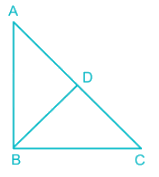½ × AC × BD = ½ × AB × BC [Equating area]
AC × BD = AB × BC
4 BD × BD = AB × BC [∵ AC = 4 BD]
Dividing by BC2 both the sides
4 × BD/BC × BD/BD = AB/BC

In triangle BDC, sin C = BD/BC and In triangle ABC, tan C = AB/BC
4sin2C = tan C
4sin2C = sinC/cos C
4sinC cos C = 1
2sin C cos C = ½
Sin2C = ½ [∵ sin 2A = 2sinA cos A]
∴ 2C = 30° and C = 15°
tan C = tan 15°
To find tan 15°
Tan 2θ = 2tanθ/ (1 – tan2 θ)
Let tan 15° be x
Tan 30° = 2x/(1 – x2)
1/√3 = 2x/(1 – x2)
1 – x2 = 2√3x
x2 + 2√3x – 1 = 0
Using quadratic formula and ignoring the negative term
x = (-2√3 + 4)/2 = 2 – √3

CDS I - Mathematics Previous Year Question Paper 2018 - Question 90

ABC is a triangle right angled at C with BC = a and AC = b. If p is the length of the perpendicular from C on AB, then which one of the following is correct?

Detailed Solution for CDS I - Mathematics Previous Year Question Paper 2018 - Question 90

Area of the triangle ABC = ½ × Base × perpendicular = ½ × Hypotenuse × Height drawn on it

½ × AC × BC = ½ × AB × p
AC × BC = AB × p
ab = AB × p
Squaring on both sides
a2b2 = AB2 × p2
AB2 = AC2 + BC2 [∵ Pythagoras theorem]
⇒ a2 + b2
∴ a2b2 = (a2 + b2) × p2

CDS I - Mathematics Previous Year Question Paper 2018 - Question 91

The radius and slant height of a right circular cone are 5 cm and 13 cm respectively. What is the volume of the cone?

Detailed Solution for CDS I - Mathematics Previous Year Question Paper 2018 - Question 91

In a cone h = √(l2 – r2) = √(132 – 52) = 12cm
Volume of the cone = 1/3 × π r2 h
⇒ 1/3 × π × 52 × 12
⇒ 100π cm3

CDS I - Mathematics Previous Year Question Paper 2018 - Question 92

Two equal circular region of greatest possible area are cut off from a given circular sheet of area A. What is the remaining area of the sheet?

Detailed Solution for CDS I - Mathematics Previous Year Question Paper 2018 - Question 92

The greatest possible area is cut off,

∴ Both the circles touch each other and extend to the two ends of the diameter of the main circle

Sum of diameters of the circle = Diameter of the larger circle

2 × diameter of the small circle = Diameter of the larger circle

The radius of the small circle = ½ × Radius of the larger circle

Area of small circle/Area of large circle = (Radius of small circle/Radius of a large circle)2

?/A = (½)2

∴ Area of small circle = A/4

Remaining area = Area of the large circle – 2 × Area of the small circle

⇒ A – 2 × A/4 = A/2

CDS I - Mathematics Previous Year Question Paper 2018 - Question 93

If the ratio of the radius of the base of a right circular cone to its slant height is 1∶ 3, what is the ratio of the total surface area to the curved surface area?

Detailed Solution for CDS I - Mathematics Previous Year Question Paper 2018 - Question 93

TSA = πr (l + r) whereas CSA = πrl
TSA/CSA = [πr (l + r)] / (πrl)
⇒ (l + r)/l
⇒ 1 + r/l = 1 + 1/3 = 4/3 [∵ r ∶ l = 1 ∶ 3]
∴ Required ratio = 4 ∶ 3

CDS I - Mathematics Previous Year Question Paper 2018 - Question 94

A right circular cone is sliced into a smaller cone and a frustum of a cone by a plane perpendicular to its axis. The volume of the smaller cone and the frustum of the cone are in the ratio 64 ∶ 61. Then their curved surface areas are in the ratio

Detailed Solution for CDS I - Mathematics Previous Year Question Paper 2018 - Question 94

For same vertical angle,

Radius of small cone/Radius of large cone = Height of small cone/Height of large cone Slant height of small cone/Slant height of the large cone = k

Volume of small cone∶ Volume of frustum = 64 ∶ 61

∴ Volume small cone∶ Volume large cone = 64 ∶ (64 + 61) = 64 ∶ 125

Let the radii, height and slant heights of the small and large cones be r, h, l and R, H, L

Volume of small cone/Volume of large cone = 64/125

(1/3 π r2 h) / (1/3 π R2 H) = 64/125
(r/R)2 × (h/H) = 64/125
k2 × k = 64/125
k3 = 64/125
∴ k = 4/5

Curved surface area of small cone/Curved surface area of large cone = (π r l) / (π R L)
⇒ (r/R) × (l/L)
⇒ k × k = k2 = 16/25

Curved surface area of small cone/Curved surface area of frustum = 16/ (25 – 16) = 16/9
∴ Required ratio 16 ∶ 9

CDS I - Mathematics Previous Year Question Paper 2018 - Question 95

There are as many square centimeters in the surface area of a sphere as there are cube centimeters in its volume. What is the radius of the sphere?

Detailed Solution for CDS I - Mathematics Previous Year Question Paper 2018 - Question 95

Numerically surface area and volumes are equal
4πR2 = 4/3 π R3
1 = R/3
∴ R = 3 cm

CDS I - Mathematics Previous Year Question Paper 2018 - Question 96

The radii of two circles are 4.5cm and 3.5 cm respectively. The distance between the centres of the circles is 10 cm. What is the length of the transverse common tangent?

Detailed Solution for CDS I - Mathematics Previous Year Question Paper 2018 - Question 96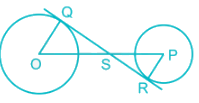In triangle OQS amd PRS
∠OQS = ∠PRS =90° [∵ Radius is perpendicular to tangent]
∠QSO = ∠PSR [∵ Vertically opposite angles are equal]
∴ OQS ∼ PRS
OQ/PR = OS/PS
4.5/3.5 = OS/PS
9/7 = OS/PS
∴ PS = 7OS/9
OP = OS + PS
10 = OS + 7OS/9
10 = 16OS/9
∴ OS = 90/16 = 5.625 cm
And PS = 10 – 5.625 = 4.375 cm
In triangle OQS, OS2 = OQ2 + QS2 [∵ Pythagoras theorem]
5.6252 = 4.52 + OS2
∴ OS = 3.375 cm
In triangle PRS, PS2 = PR2 + SP[∵ Pythagoras theorem]
4.3752 = 3.52 + SP2
∴ SP = 2.625
∴ OP = OS + SP
⇒ 3.375 + 2.625 = 6 cm

CDS I - Mathematics Previous Year Question Paper 2018 - Question 97

What is the volume of a cone of maximum volume cut out from a cube of edge 2a such that their bases are on the same plane?

Detailed Solution for CDS I - Mathematics Previous Year Question Paper 2018 - Question 97

When the cone of maximum volume cut out from cube of edge 2a, height of the cone = Edge of the cube = 2a
Radius of the cone = ½ × Edge of the cube =1/2 × 2a = a
Volume of the cone = 1/3 × π r2 h
⇒ 1/3 × π × a2 × 2a = 2πa3/3

CDS I - Mathematics Previous Year Question Paper 2018 - Question 98

A region of area A bounded by a circle C is divided into n regions, each of area A/n, by drawing circles of radii r1, r2, r3, ⋯ , rn-1 such that r < r2 < r3 < ⋯ rn-1 concentric with the circle C. If pm = rm+1 /rm, then which one of the following is correct?​

Detailed Solution for CDS I - Mathematics Previous Year Question Paper 2018 - Question 98

Area of (m + 1)th region = π (rm+12 – rm2)
⇒ πrm2 [(rm+1/rm)2 – 1]
⇒ πrm2 × (pm2 – 1)
Since area of all the region is constant
pm2 – 1 ∝ 1/rm2
So as m increases rm increases, ∴ pdecreases with the m

CDS I - Mathematics Previous Year Question Paper 2018 - Question 99

In a room whose floor is a square of side 10 m, an equilateral triangular table of side 2 m is placed. Four book shelves of size 4 m × 1 m × 9 m are also placed in the room. If the half of the rest of the area in the room is to be carpeted at the rate of Rs 100 per square meter, what is the cost of carpeting (approximately)?

Detailed Solution for CDS I - Mathematics Previous Year Question Paper 2018 - Question 99

Area to be carpeted = ½ × (Area of the square floor – Area of the equilateral triangle – 4 × Area of book shelves)
⇒ ½ × (side2 – √3/4 × side2 – 4 × l × b)
⇒ ½ × (102 – √3/4 × 22 – 4 × 4 × 1)
⇒ ½ × (100 – √3 – 16)
√3 ≈ 1.732
∴ Area to be carpeted = 41.134 m2
Cost = 41.134 × 100 ≈ Rs 4113

CDS I - Mathematics Previous Year Question Paper 2018 - Question 100

The length of a line segment AB is 2 cm. It is divided into two parts at a point C such that AC2 = AB × CB. What is the length of CB?

Detailed Solution for CDS I - Mathematics Previous Year Question Paper 2018 - Question 100

Let the length of CB be x
∴ AC = AB – CB = 2 – x
AC2 = AB × C
(2 – x)2 = 2x
4 – 4x + x2 = 2x
x2 – 6x + 4 = 0
Using quadratic formula to solve and ignoring the root greater than 2
x = (6 – 2√5)/2 = 3 – √5 cm

## CDS ( Combined Defence Services ) Mock Test Series

23 docs|33 tests
Information about CDS I - Mathematics Previous Year Question Paper 2018 Page
In this test you can find the Exam questions for CDS I - Mathematics Previous Year Question Paper 2018 solved & explained in the simplest way possible. Besides giving Questions and answers for CDS I - Mathematics Previous Year Question Paper 2018, EduRev gives you an ample number of Online tests for practice

## CDS ( Combined Defence Services ) Mock Test Series

23 docs|33 tests(Scan QR code)